To Find Different Gravity Law Proof Gravity Wave Equations are Real and Generate Gravitational Waves | OMICS International
Journal of Physical Mathematics

# To Find Different Gravity Law Proof Gravity Wave Equations are Real and Generate Gravitational Waves

Morningstar Applied Physics, LLC Vienna, VA 22182, USA

*Corresponding Author:
Morningstar Applied Physics
LLC Vienna, VA 22182, USA
Tel: 21564644
E-mail: [email protected]

Received Date: March 06, 2017; Accepted Date: April 25, 2017; Published Date: April 28, 2017

Citation: Murad PA (2017) To Find Different Gravity Laws… Proof Gravity Wave Equations are Real and Generate Gravitational Waves. J Phys Math 8: 223. doi:10.4172/2090-0902.1000223

Visit for more related articles at Journal of Physical Mathematics

#### Abstract

Various gravitational laws are examined. Binary pulsars imply unusual behavior as evidence for gravitational waves in contrast to Newtonian gravity. Gravity's intensity in the restricted three-body problem can significantly be reduced due to rotational centrifugal forces to explain shortcomings usually mentioned to invent dark matter. Although Libration Points are distinct, they represent a suitable testing ground. The Trojan asteroids at the Sun-Jupiter stable triangular points do not demonstrate that millions of asteroids reside at a singular point but with a very large scatter and do not asymptotically congeal due to attraction to create planetoids thereby suggesting Newtonian gravitation is not ample and has flaws. A phase-space trajectory methodology for an integral equation defines eigenvalues and a forcing function to test some different gravity laws. These findings indicate that a time-dependent law may not locally conserve energy and result in a celestial vibration, repulsive gravitation or produce gravitational waves. This provides insights for newer gravitational models possible suitable for using warp drive concepts. Furthermore, this effort warrants placing more space probes at the triangular Libration Points as cosmic anomalies.

#### Keywords

Pulsars; Gravity; Gravitation; Space; Time

#### Introduction

There are several different gravitational laws , which may exist for different applications and regions. The question is to find a suitable test to investigate if these models are correct or not. This may or may not require investigating anomalies. Moreover, if successfully tested, could a future model be created that possesses more granularity than an existing model with higher orbit accuracy?

Iorio  provides an excellent examination for defining specific anomalies, which are worth mentioning. There are currently accepted laws of gravitation applied to known bodies which may potentially pave the way for remarkable advances in fundamental physics. This is particularly important given where most of the Universe seems to be made of unknown substances dubbed Dark Matter and Dark Energy rather than pursuing a different model to validate a newer gravitational law other than Newtonian gravitation. Moreover, investigations can serendipitously enrich and find other solutions as well as other anomalies. The current status of some of these alleged gravitational anomalies in the Solar system are:

• Possible anomalous advances of planetary perihelia,

• Unexplained orbital residuals of a recently discovered moon of Uranus (Mab),

• The lingering unexplained secular increase of the eccentricity of the orbit of the Moon,

• The so-called Faint Young Sun Paradox,

• The secular decrease of the mass parameter of the Sun,

• The Flyby Anomaly,

• The Pioneer Anomaly, and

• The anomalous secular increase of the astronomical unit.

Obviously Newtonian gravitation is the most popular model and has worked exceedingly well while estimating orbits in the near-abroad for planets, moons, asteroids, and short-and long-range satellites. Its flaws, however, is if Einstein is correct and gravitational waves exist, Newtonian gravity is predicted by an elliptical partial differential equation and does not admit gravitational waves. This is problematic. Moreover, Einstein and other models would require time which satisfies a wave partial differential equation for the existence of gravitational waves. Current thinking of these separate models is they should all have to asymptotically satisfy the far-field boundary conditions based upon Newtonian gravitation. That is gravity should vanish at infinity. This immediately suggests the model should have some form of an inverse radial function with distance anchored at a celestial mass to satisfy this far boundary condition.

An existence of gravitational waves may not be as prevalent as one would assume. Gravitational waves are a means for allowing cosmic events to occur with some time delay as well as prevent the instantaneous responses whenever a supernova explodes. Einstein suggested there should be a time delay in such events. He attempted to generate a gravity model  with gravitational waves. In his initial model, the conclusion was gravity would be self-sustained and selffeeding. Hence gravity depends upon itself. Einstein felt this was not acceptable and changed his view to determining a geometric representation of the space-time continuum and gravity.

The other point worth mentioning is wave equations mathematically have real characteristics in their partial differential equation. If these characteristics with a hyperbolic partial differential equation, or a wave equation, confluence and join, we may have a situation analogous to fluid dynamics with respect to gravity. That is gravitational waves or gravitational shocks  may exist and can potentially use a propulsor to harness thrust for missions to the far-abroad. Thus this can become a crucial issue.

The question is why would we look at the Libration Points which have historically survived for a very long period of time? This is a reasonable request. Euler first made several contributions to include fluid dynamics. These involved using fluid particles in a stationary coordinate system in a control volume where you cannot differentiate about specific particles as well as look at fluid dynamic problems without the shear stress terms intrinsic to the Navier-Stokes equations. From an astrodynamic perspective, the Euler problem also involves the ‘restricted’ three-body problem. The rationale is when you compare celestial bodies and a space probe, the weight of the probe likened to the celestial bodies is insignificant in the order of the mass of the probe about 10-22 compared with the celestial body weights. Euler  simplified mathematically complex problems, which would defy solutions during the 18th century. This is understandable to rationally linearize nonlinear problems to gain some plausible insights.

This is very unusual in Figure 1. It shows the strength of the gravitational field of two celestial bodies. However, the important note is the effects of the coordinate system’s rotation which has an overriding consequence when the combined effects are included. One of the arguments about dark matter is where galaxy spirals did not respond as expected. Either the gravitational law is not ample or there is a need for black or dark matter. As this figure shows, without understanding rotational effects, gravity can easily be altered. Moreover, Winterberg implies that the rotation of Neutron stars can alter its gravitational field with respect to its companion in binary pulsars. Thus we need to look at rotation between celestial bodies as well as body rotation effects from frame-dragging.

Figure 1: The three-body problem can be visualized as a combination of the potentials due to the gravity of the two primary bodies along with the centrifugal effect from their rotation. Note gravitation by itself does not respond due to these significant rotational effects.

The Lagrangian or Libration Points are normally mentioned in a Cartesian Coordinate system rather than a polar coordinate system [If a polar coordinate system is used for a two-body problem, the probe or second body sees a radial attraction due to gravity from the celestial body. There is no further force that would impact the angular momentum around the primary body and the problem is solved resulting in an elliptic, hyperbolic or parabolic trajectory. The problem is straightforward. For a three-body system, the probe body sees two attractions from the primaries' gravitation. However, the angular force does not vanish with a constant such as r2dθ/dt is constant as in the two-body problem. The mathematics is complex depending upon the geometric relation with the both primaries. This in polar coordinates creates an obvious problem] to reduce difficult geometric circumstances. Motion between two large celestial bodies and the probe are confined to a two-dimensional plane. These are in a rotating coordinate system where a probe is not only influenced by the gravitational attractions for two bodies but also the centrifugal effect due to rotation about the coordinate system reference point. The origin reference point is the Barycenter of the two large primaries.

The other part of the Cartesian coordinate problem is with all of the solutions, the analysis comes down to 5 distinct points; three collinear points falling on a line joining the two primaries and two triangular points that are off to the left and to the right side of this line within a circle based upon a radius from the distance between the two primaries. One of the Libration Points falls on the same or close radius. This latter point about the collinear point is surprising and motivates to look at this problem purely with polar coordinates. The difference from this location would be dependent upon the mass ratios between the weights of the celestial primaries. Triangular points are considered stable points while the collinear points are considered as unstable. These points are selected based upon a point in the field where the probe’s gravitational and centrifugal forces possess no force in the field. This is generated in a gravitational potential combined with rotational effects. The first derivative of this potential vanishes. What is not normally considered is the stability of the five points, which h can be determined by the Calculus of Variations. Here, the force field will be stable when the field is a relative minimum and unstable when it is a relative maximum. This requires dealing with second derivatives of the field as well as set the first derivatives to zero explained in Figures 1 and 2.

Figure 2: The three collinear Lagrange points (L1, L2, and L3) were discovered by Leonhard Euler a few years before Lagrange discovered the remaining two triangular points . The gravity wells or slight mounds at the Libration Points are not seen here.

The question about different gravitational laws needs some insights. Newtonian gravitation is based upon the distance between two attractive bodies. Jefimenko [6,7] implies the two bodies are attractive but gravity is also a function of velocity to create both an attractive force as well as generate angular momentum. Jefimenko not only defines a gravity law which asymptotically satisfies Newtonian gravity but supposedly includes the effects from Einstein’s Theory of Relativity. To meet these requirements, he generates a co-gravity field to compensate for motion at speeds in the region of near and above the speed of light. Unfortunately, we are now entering a dangerous realm where we lack meaningful data or evidence to either clearly validate or deny any of these theories and models. Furthermore, according to Jefimenko, Newtonian is not a consequence of frame dragging, which includes tidal locking. This suggests that on a major planet, you will always see the same face of a large orbiting moon. If a wave equation is included in the model, gravity should also be a function of time. This requires some thought process to realize gravity is related in some manner with time. Moreover, the functional representation will involve the spatial coordinates as well as a function of time. How would this influence the mechanics in examining trajectories or relating energy that would normally accept as a constant but now would become a function of time? This suggests energy may either increase or decrease with time without any other factors which impact the dynamics. This will require some deeper consideration to really understand this point because it appears to violate conservation of momentum as well as energy. The question remains on how to define and validate a gravity law. This will have to be performed in the context of contemporary technology with some evidence. As an approach, the Libration Points will be examined from energy requirements followed by examining some trajectory performance for different gravitational laws. However, these Libration Points may provide evidence of the validity of one gravitational law over another.

#### Discussion

The question is why we should be concerned about the Libration Points. Basically, these locations using Newtonian gravitation imply that distinct but weak points exist. It is our intention to mention that triangular Libration points should also be considered as cosmic anomalies as the previously mentioned topics. Triangular Libration points were originally discovered based upon the Trojan asteroids considering the Sun-Jupiter system.

#### Data about the Trojan Asteroids

The term "Trojan" originally referred to the "Trojan asteroids" (Jupiter Trojans), which orbit close to the Lagrangian points of the Sun- Jupiter system. These were named after characters from the Trojan War of Greek mythology. By convention, the asteroids orbiting near the L4 point of Jupiter are named after the characters from the Greek side of the war, whereas those orbiting near the L5 of Jupiter are from the Trojan side. Later on, objects were found orbiting near the Lagrangian points of Neptune, Mars, Earth, and Uranus with respect to the Sun. Minor planets at the Lagrangian points of planets other than Jupiter may be called Lagrangian minor planets.

The current Trojan asteroids [8-16] are shown with a debris cloud between Mars and Jupiter’s orbits: the L4 swarm is believed to hold between 160,000 and 240,000 asteroids with diameters larger than 2 km and about 600,000 with diameters larger than 1 km. If the L5 swarm contains a comparable number of objects, there is more than 1 million Jupiter Trojans 1 km in size or larger. For the objects brighter than absolute magnitude 9.0, the population is probably complete. These numbers are similar to comparable asteroids in the asteroid belt. The total mass of the Jupiter Trojans is estimated to be low at 0.0001 of the mass of Earth or one-fifth of the mass of the asteroid belt [By contrast, the debris orbit between Mars and Jupiter has a weight, if all summed up, would be the size of the planet Mars. Thus these are relatively light and should be strongly influenced by large celestial bodies]. The number of Jupiter Trojans observed in the L4 swarm is slightly larger than observed in L5. However, because the brightest Jupiter Trojans show little variation in numbers between the two populations, this disparity is probably due to observational bias. However, some models indicate the L4 swarm may be slightly more stable than the L5 swarm. Jupiter Trojans have orbits with radii between 5.05 and 5.35 AU from the Sun (the mean semi-major axis is 5.2 ± 0.15 AU), and are distributed throughout elongated, curved regions around the two Lagrangian points. Each swarm stretches for about 26° along the orbit of Jupiter, amounting to a total distance of about 2.5 AU. Jupiter Trojans do not maintain a fixed separation from Jupiter. They slowly librate around their respective equilibrium points, periodically moving closer to Jupiter or moving farther from it. Also note there is a collection of asteroids near L3, which is an unstable Libration Point. The scatter at this location is as bad as the scatter from the L4 and L5 points which are supposedly stable. One wonders if the L1 point has a similar scatter. Astronomers have found a Trojan-like asteroid, not far from the Earth , moving in the same orbit around the Sun. It sits in one of the 'Lagrange points', which are 60 degrees ahead of or behind the planets in their orbits. These are points of gravitational stability. Called 2010 TK7, the rock is about 80 million km from Earth and should come no closer than about 25 million km. It is suggested its orbit appears stable at least for the next 10,000 years. Carefully observe the magnitude of this exceptionally large motion. There is another point worth noting. These Trojan asteroids, as well as the orbital debris cloud between Mars and Jupiter, appear to be continually moving and not having confluence with each other to form planetoids. This movement may represent a transient change in the local gravitational field. These effects should be used to account for a gravitational model. Although the motion of the asteroids may be small, this should appear as a celestial vibration. Thus the movement from these asteroids should generate high-frequency gravitational waves (HFGWs). This point is also the same with the rings around Jupiter and Saturn. Here, there is asteroid debris moving in a specific trajectory. However, amongst the debris, there is an instantaneous motion that is not continuous and the bodies obviously collide within these belts. This difference in motion, as well as collisions, should be ample to also generate HFGWs. The issue for detection should be more severe because of the presence of a large celestial body.

This could create additional noise as well as alter the HFGWs. A cleaner detection would most likely occur with the Trojan asteroids previously mentioned. The prime ingredient is toward developing a reasonable test model to examine different gravitational laws. Obviously, this pictorial in Figure 3 implies the asteroids, at the stable triangular point with a huge scatter. Moreover, the motion is quite large about these points and again, there is generally no previously mentioned confluence of the asteroids to generate planetoids based purely upon mutual attraction at any of these Libration Points whether stable or unstable. The implication is that there is sufficient movement underway to prevent the mutual gravitational attraction through some yet to be determined repulsion or another phenomenon. Some comments suggest a different law would compensate for all of these differences explained in Figure 3.

Figure 3: Estimates of the total number of Jupiter Trojans are based on deep surveys of limited areas of the sky. The false-color picture gives a better depiction of the Trojan asteroids near Jupiter’s orbit and the asteroid belt.

Demonstrating the instability in the collinear points where a probe moves from one unstable collinear point to another for the Earth- Moon system is explained in Figure 4. If the triangular points were stable, one would supposedly assume the probe may capture the probe. This surprisingly does not occur. If anything, the motion is not near the triangular points but attracted to the gravitational pull of the Moon. This suggests the Libration Points are very, very weak and not as strong as, say the celestial bodies regardless of the rotating coordinate system.

Figure 4: These results show the movement from the unstable Libration Points to each other. If stability and the influence were so strong, one would have assumed the craft would move to the triangular points. The issue is these locations are not very strong because of other to be determined effects.

#### Gravitational Models

Based upon the questions about the stability, wide scatter, and strength of the Libration Points, it would be reasonable to open the door to look at a different gravitational model from the conventional Newtonian gravity model.

There are many types of gravity laws explained in Figure 4. Basically, the Newtonian gravity law assumes there is an attraction that acts between two separate bodies. It is based on the masses of the two bodies and the separation distance between these bodies . The gravitational field acting on one of the bodies is related to the mass of the bodies as well: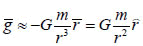(1)

Of these, r is the distance as a vector between both bodies and the ‘normal’ vector r is in the radial unit direction, G is a constant value and m is the mass. In this model, the gravity asymptotically goes to zero at extremely large distances or disappears at infinity. Some of these laws are briefly described below. Winterberg’s rule accounts for situations with pulsars and neutron stars. This implies the large rotational rate of these bodies somewhat change the strength of the gravitational attraction. Thus there is a consideration for angular momentum in the body itself as a source term. Winterberg does not prove this rationale and differences are observed between the companion star and the neutron star in binary pulsars. This difference in mass could be attributed as a similar effect as dark matter. Four-derivative theories [19,20] are a conformal gravity as an example from the theory of relativity. This means each term in the wave equation can contain up to 4 derivatives. There are pros and cons of 4-derivative theories. The pros are that the quantized version of the theory is more convergent and renormalisable. The cons are there may be issues with causality. A simpler example of a 4-derivative wave equation is the scalar 4-derivative wave equation: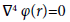(2)

This in a central field of force is explained through Table 1. The first two terms are the same as a normal wave Equation. Since this equation is a simpler approximation to conformal gravity, then m corresponds to a mass of the central source. The last two terms are unique to 4-derivative wave equations. It was suggested to assign small values to them to account for the galactic acceleration constant (also known as dark matter) and the dark energy constant. An equivalent solution to the Schwarzschild solution in General Relativity on a spherical source for conformal gravity has a metric with:

Gravity law Assumptions Gravitational rule
Newtonian gravitation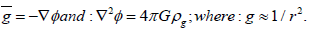Four-Derivative theories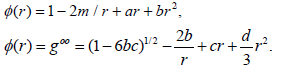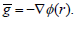Winterberg’s rule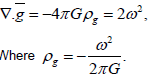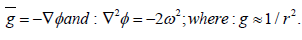Jefimenko’s gravity and co-gravity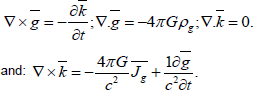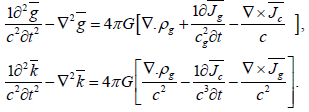Murad’s modification of Jefimenko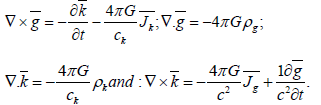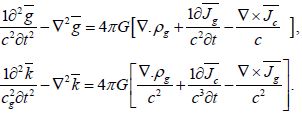Murad’sgravity law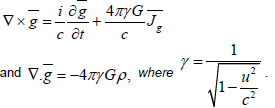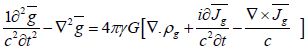Table 1: Different Gravitational Laws which cover a spectrum of conditions of interest.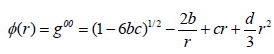(3)

The term 6bc is very small so it can be ignored. The problem is that now c is the total mass-energy of the source, b is the integral of density time’s distance to the source squared. So this is a completely different potential to General Relativity and not just a small modification. The main issue with conformal gravity theories, as well as any theory with higher derivatives, is the typical presence of instabilities of the quantum version of the theory, although there might be a solution to this problem. Note these theories do not approach to Newtonian gravitation because of the r and r2 terms. However, based on establishing different views about results during Pioneer 10 and 11, the existence of such terms may be explained. Iorio  performs an adequate definition where the attraction of the sun’s gravity at a certain threshold distance becomes linear and a constant similar to these terms estimated at very small values. The Jefimenko gravitational model involves an attractive force between two bodies as well as creates angular momentum. The gravitational law is not only a function of distance between the two bodies but also includes the relativity velocity function between the two bodies. The Jefimenko model involves: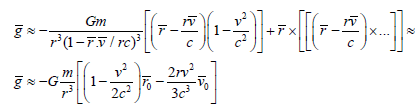(4)

The last two laws in Table 1 are modifications to Jefimenko’s laws from the author. The point here in the first system of partial differential equations is to obtain symmetry between the gravitational and co-gravitational fields. Whereas Jefimenko considers gravitational currents, the law considers that co-gravitational currents should also exist. The same holds for the co-gravity source term yet to be defined. A discipline of interest would be to examine the creation and experimental evidence of any of these currents and sources. The last law is an attempt to use this logic without co-gravitation. Mathematically, this involves using an imaginary term. One may hypothesize this as possibly representing an unknown dimension. Although it satisfies a wave equation and satisfying the Newtonian gravitational law, it would be considered as an improbable situation.

Test models

A reasonable test would involve specific regions that possess unusual trajectory performance or even result in singularities [21,22]. For example, the Libration Points are derived from a possible singularity region in the phase-space representation of the ‘restricted’ three-body problem. These points indicate that gravity attractions between the two rotating bodies compensate for each other as well as the motion of the third body. If a singularity, it would become infinite such as dividing by a distance where it goes to zero. Thus Libration or Lagrangian points would be interesting for testing different gravity models. We have to raise the question where there are five Libration Points using Newtonian gravitation. Countless analyses have looked at these points and others have assessed analytical means to generate enough stability to deal with these unstable points. The major issue, however, is that very few satellites or space crafts have really reached these locations. Moreover, valid models may have more of these points.

Analysis

This section will cover the conventional wisdom as well as variations which impact orbital trajectories or singular-like behavior. Again, the objective is to find plausible trends but not necessarily uncover specific results.

Preliminary results for orbits and trajectories

Let us consider a two-body problem using Newtonian gravity. With these assumptions, the radial and angular momentum equation are defined as: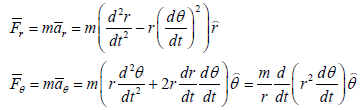(5)

Subscripts for the forces in the LHS are not derivatives but actually the radial and azimuthal force directions respectively. Derivatives are functions of time. The radial force is based upon the gravitational attraction from the larger body. Moreover, the second equation assumes the azimuthal force vanishes for each of these bodies. The radial dimension is changed as the difference between the distances to the two objects. The problem is reduced to one free-variable with some definitions where μ is the total mass of both bodies (G (m1+m2)). This is considered as the gravitational attraction for this problem as follows: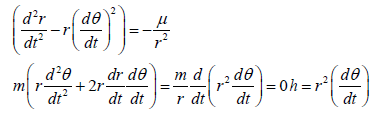(6)

Clearly, the azimuthal gravitation disappears with the constant, h, that is the angular momentum per unit mass used to satisfy azimuthal acceleration. Thus, the second equation vanishes. A variable u is selected based upon an inverse function of the radius to simplify the problem: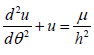(7)

The solution of this ordinary differential equation considering a geometric length l and eccentricity e is: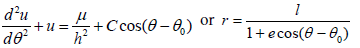(8)

Jefimenko’s two-body problem

By applying the gravitational law, the effects of gravity will affect both the radial and angular momentum equations which become: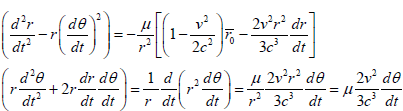(9)

Let velocity v of the spacecraft be assumed, albeit a bad assumption, as a constant. This is not as simple to alter the time derivative for angular changes. This would derive a relationship for the radial derivatives and a possible solution. First, find a solution by defining angular velocity: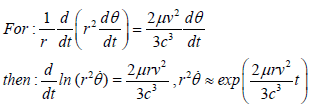(10)

Under restrictive assumptions, angular momentum is a function of the radius and time in a very small exponential term. Moreover, this exponential term may go to zero and this reverts to the Newtonian angular momentum case. However, if true, there is still an additional term in the radial momentum as an insignificant number. Furthermore, angular momentum can be altered as a function of time influencing trajectories. Note further the value of the exponential term may be close to zero for slow speeds analogous to the initial problem. It is interesting angular momentum is also a function of the rate of change of the angle. In a circular trajectory, this would be a constant term. However, for an elliptical orbit, this value changes based upon its location within the orbit. Unfortunately, there is no simple way to convert time into an angular function for this law. There is another option. Let us assume the angular momentum can be a function of the angle. This would include the terms in such expressions as in Jefimenko. For the simplest case, let: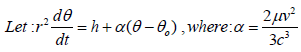(11)

Note the value of α is still an extremely small value because of the denominator. Using the inverse function for the distance r for u, these equations become: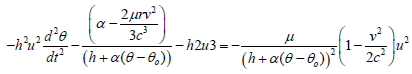(12)

Simplifying this becomes: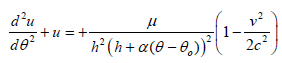(13)

This is a nonlinear equation because of the denominator in the RHS. One possible solution is to use the Green’s function with the initial value problem [15,16]. The solution becomes: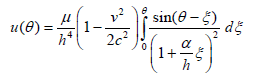(14)

Gravitational hyperbolic partial differential equation Model

Einstein’s theory of relativity implies the gravitational model satisfy wave equations, say, such as: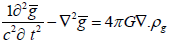(15)

If you look at the separation of variables, the homogeneous equation provides some variables to represent a function of time as well as the radial distance. The time term looks like:

T(t)=α12 t+α3 cosh(λ2t)+α4 sinh(λ2t)+α5 cos(ωt)+α6 sin(ωt), for g(t,r)= T(t). R(r) (16)

These constants make non-dimensional values by initial or final conditions. The issue is how Newtonian gravitation can be correct without considering the time factors. Constants, either λ2 orω, depend upon real values. However, the hyperbolic sine and cosine terms are never observed since they most likely occur early during gravitational creation. This is not trivial. Moreover, the two functions asymptotically are large values as an exponential function of time and may approach a line that could cancel out the t term. This may be related to the coefficients α3 if it is equal-α4, where these two terms would vanish at large time values with:

T(t)=α12 t+α3 cosh(λ2t)(1-tanh((λ2t))+α5 cos(ωt)+α6 sin(ωt) (17)

The sinusoidal and cosine terms rise and fall with time while the coefficient of the cosh term will vanish after some considerable time. This implies the hyperbolic terms at zero time would have some value suggesting gravity initial exists, and then slowly decays. Here this initial value could be cancelled out by the α1 term to compensate for this initial value unless gravity always existed before the Big Bang. However, these transient terms in this equation also imply several interesting features worth noting. These relations allow for the presence of sinusoidal behaviour. When we do this, we feel the magnitude of the constant factor α1, is large with the coefficient for the sinusoidal term. If this second term is greater than the constant, it might lead to positive gravity or repulsion. The presence of these transient terms may explain why there is such a large variation in the Trojan Asteroids near the triangular Libration Points. The geometric functions indicate gravitational waves exist and may be of very small magnitudes. Values for α1 may approach a negative unity while the α2 constant may be very small. The two-body problem modified for a wave equation now would add a term similar to: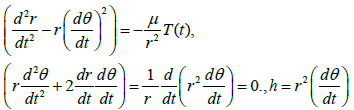(18)

Here, there is no additional complexity for the angular momentum but only the transient radial momentum effects seen. This is an interesting point. For example, many planets and binary pulsars such as 1913+16  have unusual rotation about the primary orbits. This may increase as a function of time. Here the latter example indicates the unusual trajectory motion is caused by the loss of energy in the neutron star generating gravitational waves. Motion is dominated by initial energy levels to define specific trajectories of the asteroids illustrated in Figure 5. The issue is how to relate time to spatial coordinates and especially angular changes. This is something which will not happen at the current time period. The only point is a comment made by Kozyrev who said the sun is not a thermonuclear fusion device because it should possess a higher surface temperature. When asked, what is a star? His response was: a star is a machine that converts space-time continuum into energy! Mass converting into energy, but how can we relate time to either angular momentum, energy or for that matter, mass? This is beyond our realm of technology but it deserves to raise the issue. Furthermore, transient terms suggest asteroids or other bodies near a Libration Point will be like a pot of boiling water always changing and altering the energy probably within the ZPE.

Preliminary results for Libration points

Examine the restricted three-body problem and look at finding the Libration Points using Newtonian Gravitation. For the threedimensional Restricted Three-body problem, the kinematics are: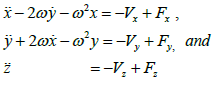(19)

The force F terms will be ignored; the dot term signifies time derivatives; where x, y, and z are elements of a Cartesian coordinate system, ω is the rotational rate, and the gravitational law requires: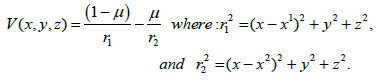(20)

The value r is the distance between the major bodies based upon the subscript and the third body, μ is the normalized weight of the larger bodies. The energy expression is found by multiplying the first equation on x with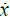integrated in time. This is achieved with y multiplied by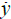and z by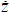integrated as a function of time. Libration points are based upon when the derivatives of V vanish. The resulting energy equation is: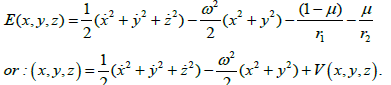(21)

Time does not appear explicitly. However, time may change related to variations with any of the other terms. For example, if gravity changes with time, then energy would also be a function of time. However, the question is where the energy comes from considering conservation. What does this mean? If unstable, a satellite placed at the collinear points would require some station-keeping propulsion to maintain its location whereas the stable points should require lower propulsion needs. The problem for Jefimenko’s law as follows: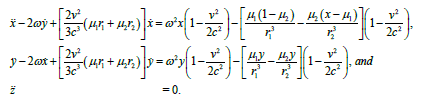(22)

A correction term is (1 – v2/c2). This is a very complicated situation with additional cross-coupling terms. Finding the energy term is difficult and finding the actual Libration Points. If the gravitational law involves a wave equation, a similar correction term is used as a function of time. The gravitational gradient looks like: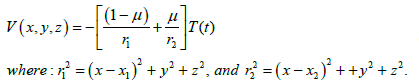(23)

Energy becomes: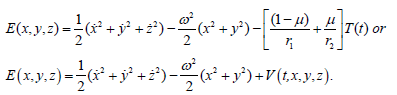(24)

In both of these cases, the energy is no longer a constant but includes a correction factor for the speed of light in the first state and a function of time in the second situation. This latter situation could explain why the collinear points are unstable requiring thrust for station keeping. Likewise, the speed of light factor suggests if a spacecraft increases, the influence of the primary bodies’ gravity diminishes and has less of a navigation problem for influencing the spacecraft. Clearly, we have two different possible outcomes with contrasting views based upon the speed of the spacecraft. This may be ideal for a test function. For example, more knowledge needs to be found regarding the dynamics at these Libration Points to see if these additional terms are real or not. If not, then this gravitational law should be considered as invalid. Furthermore, the sense of energy varying as a function of time-based upon its influence needs to be confirmed. If not, one might jump to the conclusion equations are not valid and the gravity model for the Theory of Relativity may warrant some further investigations.

Libration points

The five Libration Points are found at the location where the effects from the primary bodies and the centrifugal motion are examined such that the attractions at the probe vanish where the first derivatives of the gravitational potential V(x, y, z) vanishes. Considering the time effect in V (t, x, y, z), you essentially have the same five points but there is an addition based upon the time factor for the wave equation. This would be assumed as a sinusoidal type of change and should alter these locations. One could argue the reason for the unstable points is because they significantly move as a function of time. Similar comments are valid with the stable points.

For Jefimenko’s law, which already satisfies a wave equation, there is an additional time term which includes an effect with velocity. Hence, the other transient terms are already buried in the other expressions in lieu of V. Thus, it warrants placing a probe at these locations and notes what occurs at these trajectories. There would be two separate issues. This involves first the motion and/or location of these points and then the impact of how the trajectories would be changed based purely on the gravitational law.

Phase space representation of the restricted three-body problem

The issue is how the probe’s trajectory would be altered especially near the Libration Points. If chosen correctly the three-body problem can be formulated with a linear matrix as a viable solution. The approach is to look at some different gravitational laws based upon changing the eigenvalue solution or inclusion of a forcing function. This will provide some insights. A phase-space representation using eqn. (19), the equations of motion are: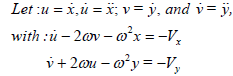(25)

These equations are rewritten as: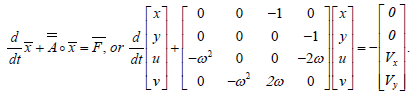(26)

Four eigen values for the A matrix are λi= ± ω, ± ω; this includes repeated eigenvalues. The reason that the eigen value is important, as well as the forcing function, is that you are looking at a trajectory similar to: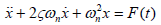(27)

Thus, by noting the differences in the frequency and the damping factor, different responses should be seen from those with different factors. However, in this problem, there is no damping function. Thus we need to establish the eigenvalues and the forcing functions, which would result in different trajectories. The solution to this problem is: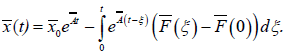(28)

Initial conditions are included with the forcing function at zero time, which may not be zero. The exponential matrix is based on the eigenvalues such as: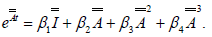(29)

One may replace the matrix with the eigenvalues. The identity matrix is the first term and there are four equations to be found. The values are: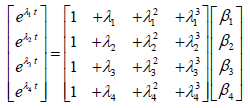(30)

The βi terms are found by using the inverse matrix. Clearly, this logic demonstrates the impact of the eigenvalues λi as well as the forcing function F upon the solution of the probe’s eventual trajectory. With repeated eigenvalues, this yields: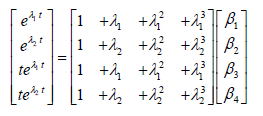(31)

Note the effects of the repeated eigenvalues that can be expected with the value of the inverse matrix. This solution is a time of function in lieu of an angle orientation as would normally be considered for the two-body problem. Also, the exponential matrix provides a sinusoidal-like function of time multiplied by time, which should be very interesting.

Wave gravitational equation: The impact of involving a wave equation for gravity affects the forcing function as a time function. Here the equation used will look like: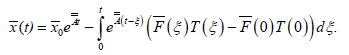(32)

This solution does not impact the eigenvalues or the basic nature of the types of trajectories involved. However, time will alter trajectories and implies the Libration Point locations will be different.

Jefimenko gravitational law: Extra terms using velocity with the correction speed of light factor is intrinsic to this law. The equations change in: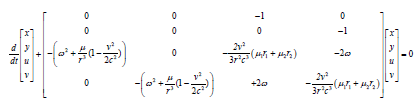(33)

The distance r might be confusing also recall r1 and r2 are functions of x and y. In this, r may be the distance of the probe measured from the barycentre of the two primaries. Despite this, there are many problems with this equation. The solution is not the same due to nonlinearity in the matrix with the distances from the probe to the two primaries as a function of both x and y. Hence the nonlinear effect exists. Moreover, this does not provide the solution previously mentioned. Despite this vector equation, we can make some judgments regarding a solution. The eigenvalues change from the matrix which indicates the equation will be different due to damping factors and frequency responses of the matrix. However, the coefficient for these additional terms is a very small number. If at high speed of the order of the speed of light, some terms would diminish or disappear. This trajectory as well as in the wave equation will provide notable differences compared to the Newtonian results.

If some of these variables are used as forcing functions, the matrix can be simplified as in: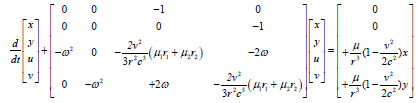(34)

Note this simplifies the eigenvalues of the previous matrix; however, there is a nonlinear forcing function in the matrix whereas it vanished in the previous matrix. Restrictive assumptions are made regarding the time factor for both matrices. Unfortunately, in this formulation, the final results cannot be established because the matrices contain functions of a radius and thus, time but all we can do is estimate trends in the eigenvalues.

#### Results

These results show some unusual trends regarding Newtonian gravitation. This is obvious because of maturity, where newer gravity relationships need to be determined. Some of this difference is the energy equations and results in either more kinematic components or time. There is a need to continue and exploit these relationships to more final conclusions. Successful results will be if Lagrangian points can be predicted, and how much of a variation exists with the Newtonian Libration Point locations. The most puzzling terms are the inclusion of time functions for wave equations and the use of a gravity model for relativity. Eqns. (15)-(18) are crucial because it demonstrates, as Jefimenko suggests, gravity includes distance as a relationship for velocity especially when using the initial-value problem with one of the boundary conditions. If there is a comparison between Jefimenko and relativity, more so than what Jefimenko claims, this use of a partial differential wave equation would be a valuable contribution.

Other functions of time should also look at eqn. (30). This may provide a different insight with gravity to integrate into space propulsion traveling with the far-reach. These are some important considerations for these situations. Although we are defining trajectories for these different gravitational laws, place the probe at the Libration Points and examine what occurs. Considerable evidence suggests there would be some variations in these locations as well as their movement as a function of time. For the wave equation, you have the same Libration Points but these points will shift as a function of time; the time factor is most likely sinusoidal with a large scatter. Thus it implies where gravity may grow and fall as a function of time. Energy might not be conserved but energy is also a function of time. Where do this energy and the momentum occur to provide conservation? In other words, an asteroid reaches the zone near the triangular point with a given initial value. The asteroid moves in locations that possess less than or equal to the energy of the asteroid. Thus the asteroid with its given energy can go into zones with lesser energy. Although the total energy may be conserved, values may change from one asteroid to another. Regarding Jefimenko’s gravity, the Libration Points may be at different locations from Newtonian gravitation.

The effect of time should also be imbedded in these results in which Jefimenko’s gravity and co-gravity equations are also wave equations supporting gravity waves. Here, there should be additional terms to include (t). Thus, these trajectories should also move about these points. Similarly there is a relationship for these equations if a relationship can be established to account for the angular rotation rate with time.

#### Conclusion

This investigation stressed if different gravitational models exist, there should be some evidence to test and validate these separate models. Some models require increasing energy as a function of time. For the Libration Points, the existence of time due to using a Relativistic model, may explain the unstable collinear points. Some solutions for simple problems create mathematical issues which do not create simple or current trivial trajectory solutions. If anything, the use of Newtonian gravitation leads toward viable and simple mathematical representations; however, if we are to treat with dark matter and the unknowns in the far-abroad to discover unusual phenomenon, will we be capable of investigating other possible models that may be required? Thus, the need for placing a probe near these points is warranted to obtain data treating these points as anomalies.

#### References

Select your language of interest to view the total content in your interested language

### Article Usage

• Total views: 1566
• [From(publication date):
June-2017 - Feb 23, 2020]
• Breakdown by view type
• HTML page views : 1432Can't read the image? click here to refresh